Download Presentation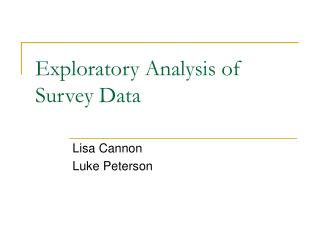Exploratory Analysis of Survey Data

# Exploratory Analysis of Survey Data - PowerPoint PPT Presentation

Exploratory Analysis of Survey Data. Lisa Cannon Luke Peterson. Presentation Outline. Density Estimation Nonparametric kernel density estimates Properties of kernel density estimators Other methods Graphical Displays NHANES data. Three features that distinguish survey data:.I am the owner, or an agent authorized to act on behalf of the owner, of the copyrighted work described.
Download Presentation## Exploratory Analysis of Survey Data

An Image/Link below is provided (as is) to download presentation

Download Policy: Content on the Website is provided to you AS IS for your information and personal use and may not be sold / licensed / shared on other websites without getting consent from its author.While downloading, if for some reason you are not able to download a presentation, the publisher may have deleted the file from their server.

- - - - - - - - - - - - - - - - - - - - - - - - - - E N D - - - - - - - - - - - - - - - - - - - - - - - - - -
Presentation Transcript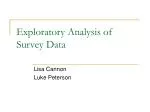### Exploratory Analysis of Survey Data

Lisa Cannon

Luke Peterson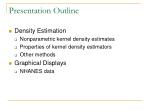Presentation Outline
• Density Estimation
• Nonparametric kernel density estimates
• Properties of kernel density estimators
• Other methods
• Graphical Displays
• NHANES data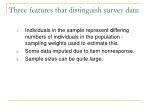Three features that distinguish survey data:
• Individuals in the sample represent differing numbers of individuals in the population - sampling weights used to estimate this.
• Some data imputed due to item nonresponse.
• Sample sizes can be quite large.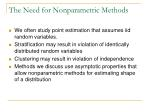The Need for Nonparametric Methods
• We often study point estimation that assumes iid random variables.
• Stratification may result in violation of identically distributed random variables
• Clustering may result in violation of independence
• Methods we discuss use asymptotic properties that allow nonparametric methods for estimating shape of a distribution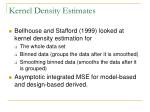Kernel Density Estimates
• Bellhouse and Stafford (1999) looked at kernel density estimation for
• The whole data set
• Binned data (groups the data after it is smoothed)
• Smoothing binned data (smooths the data after it is grouped)
• Asymptotic integrated MSE for model-based and design-based derived.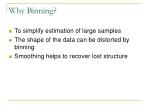Why Binning?
• To simplify estimation of large samples
• The shape of the data can be distorted by binning
• Smoothing helps to recover lost structure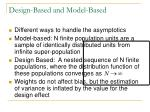Design-Based and Model-Based
• Different ways to handle the asymptotics
• Model-based: N finite population units are a sample of identically distributed units from infinite super-population
• Design Based: A nested sequence of N finite populations, where the distribution function of these populations converges as
• Weights do not affect bias, but the estimation of variance is inflated by the value for the design effect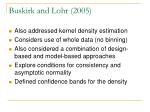Buskirk and Lohr (2005)
• Also addressed kernel density estimation
• Considers use of whole data (no binning)
• Also considered a combination of design-based and model-based approaches
• Explore conditions for consistency and asymptotic normality
• Defined confidence bands for the density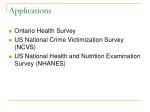Applications
• Ontario Health Survey
• US National Crime Victimization Survey (NCVS)
• US National Health and Nutrition Examination Survey (NHANES)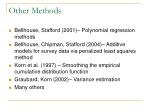Other Methods
• Bellhouse, Stafford (2001)– Polynomial regression methods
• Bellhouse, Chipman, Stafford (2004)– Additive models for survey data via penalized least squares method
• Korn et al. (1997) – Smoothing the empirical cumulative distribution function
• Graubard, Korn (2002)– Variance estimation
• Many othersPlotting Survey Data
• Common difficulties with plotting survey data:
• Dealing with sampling weights
• Plotting a large number of observations can be difficult to interpret
• See Korn and Graubard (1998).National Health and Nutrition Survey (NHANES)
• Has been conducted on a periodic basis since 1971.
• Completes about 7,000 individual interviews annually.
• Analyzes risk factor for selected diseases and conditions.
• Sample implemented is a stratified multistage design.
• Data available at http://www.cdc.gov/nhanesGlycohemoglobin Level (Ghb)
• A blood test that measures the amount of glucose bound to hemoglobin.
• Normally, about 4% to 6%.
• People with diabetes have more glycohemoglobin than normal.
• The test indicates how well diabetes has been controlled in the 2 to 3 months before the test.
• Source: http://my.webmd.comHistograms
• Histograms provide a nice summary of the distribution of large data sets.
• Suppose that we would like to assess the distribution of glycohemoglobin levels.
• Sampling weights must be considered before plotting a histogram.SAS Code: Account for Weights

proc univariate data=explore.glyco noprint;

var glyco;

freq weight;

histogram / nrows=2 cfill=red midpoints=3 to 15 by 0.5 cgrid=grayDD;

run;

• The variable weight indicates the number of population units the sample unit represents.Boxplots
• Boxplots indicate location of important summary statistics along with distribution.
• See Figures 7.8 and 7.10 in Lohr.
• The boxplot procedure in SAS will not accept any arguments to account for weights.
• The survey library in R will.Graphs for Regression – Bubble Plots
• Scatterplots are inadequate for survey data as they fail to account for sampling weights.
• Bubble plots incorporate the weights by making the area of each circle proportional to the number of population observations at those coordinates (See Lohr, Chapter 11).
• The ordinary least squares regression line is then replaced by a weighted least squares line.
• See Figure 11.5 in LohrDealing with Large Samples
• Bubble plots are hard to interpret for large data sets due to overlapping bubbles.
• Potential solutions:
• Create a “sampled scatterplot” in which we sample from the original data where probability of selection is proportional to sample weights.
• “Jitter” the data by adding some random noise to the values before plotting.
• These and others discussed in Korn and Graubard (1998).SAS Code: Plotting a representative subsample

proc surveyselect data=explore.glyco out=plotdata method=pps sampsize=300 seed=3452;

size weight;

run;

symbol1 v=circle i=r c=black ci=green w=2;

proc gplot data=plotdata;

plot glyco*age;

run;Plotting Recommendations
• For univariate displays, adjust for the sampling weights.
• For scatterplots, sampling weights can be accounted for by using bubble plots.
• If the sample is large, a subsampling procedure that incorporates the weights might be more appropriate.References
• Bellhouse ,D.R. and Starfford, J.E. (1999). Density Estimation from complex surveys. Statistica Sinica.
• Bellhouse, D. R. and Stafford, J.E. (2001). Local polynomial regression in complex surveys. Survey Methodology.
• Bellhouse, D.R. and Stafford, J.E. (2004). Additive models for survey data via penalized least squares. Technical Report.
• Buskirk, T.D. and Lohr, S.L. (2005). Asymptotic properties of kernel density estimation with complex survey data. Journal of Statistical Planning and Inference.
• Graubard, B.I. and Korn E.L. (2002). Inference for superpopulation parameters using sample surveys. Statistical Science.
• Korn, E.L., Midthune, D., and Graubard, B.I. (1997). Estimating interpoloated percentiles from grouped data with large samples. J. Official Statist.
• Korn, E.L. and Graubard, B.I. (1998). Scatterplots with survey data. The American Statistician.